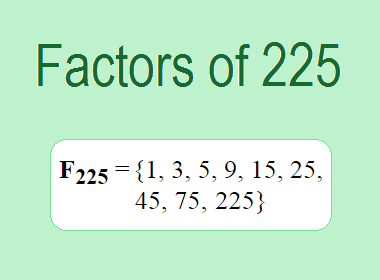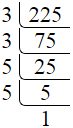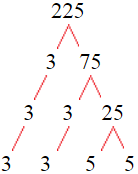# Factors of 225The factors of 225 are 1, 3, 5, 9, 15, 25, 45, 75, and 225 i.e. F225 = {1, 3, 5, 9, 15, 25, 45, 75, 225}. The factors of 225 are all the numbers that can divide 225 without leaving a remainder.

We can check if these numbers are factors of 225 by dividing 225 by each of them. If the result is a whole number, then the number is a factor of 225. Let's do this for each of the numbers listed above:

·        1 is a factor of 225 because 225 divided by 1 is 225.

·        3 is a factor of 225 because 225 divided by 3 is 75.

·        5 is a factor of 225 because 225 divided by 5 is 45.

·        9 is a factor of 225 because 225 divided by 9 is 25.

·        15 is a factor of 225 because 225 divided by 15 is 15.

·        25 is a factor of 225 because 225 divided by 25 is 9.

·        45 is a factor of 225 because 225 divided by 45 is 5.

·        75 is a factor of 225 because 225 divided by 75 is 3.

·        225 is a factor of 225 because 225 divided by 225 is 1.

## How to Find Factors of 225?

1 and the number itself are the factors of every number. So, 1 and 225 are two factors of 225. To find the other factors of 225, we can start by dividing 225 by the numbers between 1 and 225. If we divide 225 by 2, we get a remainder of 1. Therefore, 2 is not a factor of 225. If we divide 225 by 3, we get a remainder of 0. Therefore, 3 is a factor of 225.

Next, we can check if 4 is a factor of 225. If we divide 225 by 4, we get a remainder of 1. Therefore, 4 is not a factor of 225. We can continue this process for all the possible factors of 225.

Through this process, we can find that the factors of 225 are 1, 3, 5, 9, 15, 25, 45, 75, and 225. These are the only numbers that can divide 225 without leaving a remainder.

********************

********************

## Properties of the Factors of 225

The factors of 225 have some interesting properties. One of the properties is that the sum of the factors of 225 is equal to 403. We can see this by adding all the factors of 225 together:

1 + 3 + 5 + 9 + 15 + 25 + 45 + 75 + 225 = 403

Another property of the factors of 225 is that they are all odd numbers. This is because 225 is an odd number, and any even number cannot divide an odd number without leaving a remainder.

Another property of the factors of 225 is that the prime factors of 225 are 3 and 5 only.

## Applications of the Factors of 225

The factors of 225 have several applications in mathematics. One of the applications is in finding the highest common factor (HCF) of two or more numbers. The HCF is the largest factor that two or more numbers have in common. For example, to find the HCF of 225 and 135, we need to find the factors of both numbers and identify the largest factor they have in common. The factors of 225 are 1, 3, 5, 9, 15, 25, 45, 75, and 225. The factors of 135 are 1, 3, 5, 9, 15, 27, 45, and 135. The largest factor that they have in common is 45. Therefore, the HCF of 225 and 135 is 45.

Another application of the factors of 225 is in prime factorization. Prime factorization is the process of expressing a number as the product of its prime factors. The prime factors of 225 are 3 and 5, since these are the only prime numbers that can divide 225 without leaving a remainder. Therefore, we can express 225 as:

225 = 3 × 3 × 5 × 5

We can do prime factorization by division and factor tree method also. Here is the prime factorization of 225 by division method,225 = 3 × 3 × 5 × 5

Here is the prime factorization of 225 by the factor tree method,225 = 3 × 3 × 5 × 5

## Conclusion

The factors of 225 are the numbers that can divide 225 without leaving a remainder. The factors of 225 are 1, 3, 5, 9, 15, 25, 45, 75, and 225. The factors of 225 have some interesting properties, such as being odd numbers and having a sum of 403. The factors of 225 have several applications in mathematics, such as finding the highest common factor and prime factorization.